# Mixing 'Em Up

## OBJECTIVE: To learn how to add and subtract mixed numbers

In a previous lesson, you learned how to add and subtract SIMILAR and DISSIMILAR FRACTIONS. Wearing Uniforms: https://www.geogebra.org/m/ceyu3wpa In this lesson, you'll learn how to add and subtract MIXED NUMBERS. There are two common methods for adding and subtracting MIXED NUMBERS. Method 1: Change the MIXED NUMBERS to IMPROPER FRACTIONS and proceed as in adding or subtracting fractions. Examples: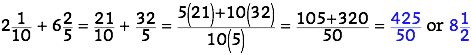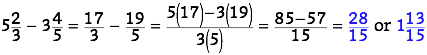Method 2: Add or subtract the whole numbers and the fractions SEPARATELY. Examples: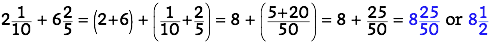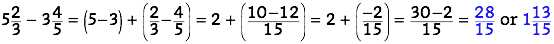The last process can be a bit tricky because you'll be combining a positive whole number and a negative fraction. A trick you can use here is the BUTTERFLY METHOD (see above link), but first you have to rewrite 2 as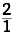.
Below is a set of problems involving adding and subtracting mixed numbers.

## In this lesson, you learned how to add and subtract mixed numbers.

In future lessons, you'll learn more about operations on fractions and mixed numbers. Did you ENJOY today's lesson?